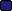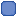###Author Topic: AGI Random Number Generator  (Read 3085 times)

0 Members and 1 Guest are viewing this topic.

####c-square

• Bug Breeder
•• Posts: 3
• 26.00 credits
• View Inventory
• Send Money To c-square
• Karma: +0/-0
• I'm a Llama!##### AGI Random Number Generator
« on: November 19, 2017, 12:38:22 AM »
Hi,

I'm trying to crack the RNG behind the slot machine in SQ1 to help someone with a TAS of the game.  We've found the code for NAGI's random function:
Code: [Select]
`       u16 diff;       u16 min;       u16 max;              min =  *(c++);       max = *(c++);       diff = max - min +1;       state.var[*(c++)] = (agi_rand() % diff) + min;       return c;`
where agi_rand() calls:

Code: [Select]
`   if (agi_rand_seed == 0)        {              //printf("Creating new randomised seed...\n");              /* ah = 0; int(1Ah); */     // number of ticks since midnight#ifndef RAD_LINUX              _ftime (&t);         // time since 1970 but that's ok#else              ftime(&t);#endif              //printf("time = %ld seconds %ld milliseconds\n", t.time, t.millitm );              agi_rand_seed = t.time * TANDY_CLOCK_PER_SEC;              agi_rand_seed += (u16) ((double)t.millitm  / 1000 * TANDY_CLOCK_PER_SEC);              //printf("seed = 0x%04X\n\n", agi_rand_seed);       }        agi_rand_seed = 0x7C4D * agi_rand_seed + 1;       r = agi_rand_seed ^ (agi_rand_seed>>8);        return( r );`
and this seems to be correct some of the time, but not always.  JAGI seems to have a completely different function.

Does anyone know how I can find out the random logic used in the actual AGI interpreter?

####lskovlun

• MT Veteran
• SCI Guru
•• Posts: 563
• 4449.00 credits
• View Inventory
• Send Money To lskovlun
• Karma: +10/-0##### Re: AGI Random Number Generator
« Reply #1 on: November 19, 2017, 01:36:24 PM »
Lance Ewing HWM and NewRisingSun dug up some authentic source code a while ago and posted it in another thread:
http://sciprogramming.com/community/index.php?topic=1418.15

It's not all there, but happily, the Random function seems to be among the scraps.
« Last Edit: November 19, 2017, 01:41:34 PM by lskovlun »

####AGKorson##### Re: AGI Random Number Generator
« Reply #2 on: November 23, 2017, 01:19:12 AM »
I've decompiled the PC versions, and the random function is the same in all of them.

random(byt LOWER, byt UPPER, var vRESULT);

A. The two values passed as LOWER and UPPER are stored on the stack. A third stack variable, DELTA is stored as UPPER-LOWER+1.

B. The AX register is loaded with a 8 bit pseudo-random number by function call rand() as follows:
-if the RNDSEED(unsigned 16 bit memory location) value is zero, it is loaded with current clock count (the DX value after a call to INT 1Ah), otherwise current RNDSEED value is used
-a constant value of 7C4Dh is multiplied (unsigned  16 bit) by the RNDSEED value; result stored as 32 bit value in DX:AX
-AX value (lower 16 bits of result) are incremented by 1
-AX is then stored as new RNDSEED value
-AL is XOR'ed with AH
-AH is cleared
-AX value returned to calling function (since AH is cleared, result is pseudo-random 8 bit unsigned integer)

C. The random value from B is divided (unsigned 16 bit) by DELTA. Quotient stored in AX, remainder stored in DX

D. Remainder (DX) moved to AX; LOWER added to AX

E. AL stored in vRESULT.

NOTES:
If DELTA is positive, result will be (pseudo)random number between (and including) LOWER and UPPER
If DELTA is zero (i.e. LOWER=UPPER+1), AGI will freeze due to attempted DIVIDE BY ZERO
If DELTA is negative, result will be (pseudo)random number between 0 and 255

The seed value (RNDSEED) is initially set using the cpu internal clock; unless you can manipulate the clock so AGI has the exact same clock count when the rand() function is called each time AGI is run, it's not possible to know the starting seed; thus the pattern of random numbers can't be guessed/calculated easily.

The AGI functions wander(obj oA) and follow.ego(obj oA, byt STEP, flg fDONE) also use the rand() function. Since these will change the seed value, even if you were able to control the initial seed value (by manipulating the internal clock) you need to make sure you account for calls to these functions as well.

NAGI is closest to the original.

Page created in 0.091 seconds with 24 queries.